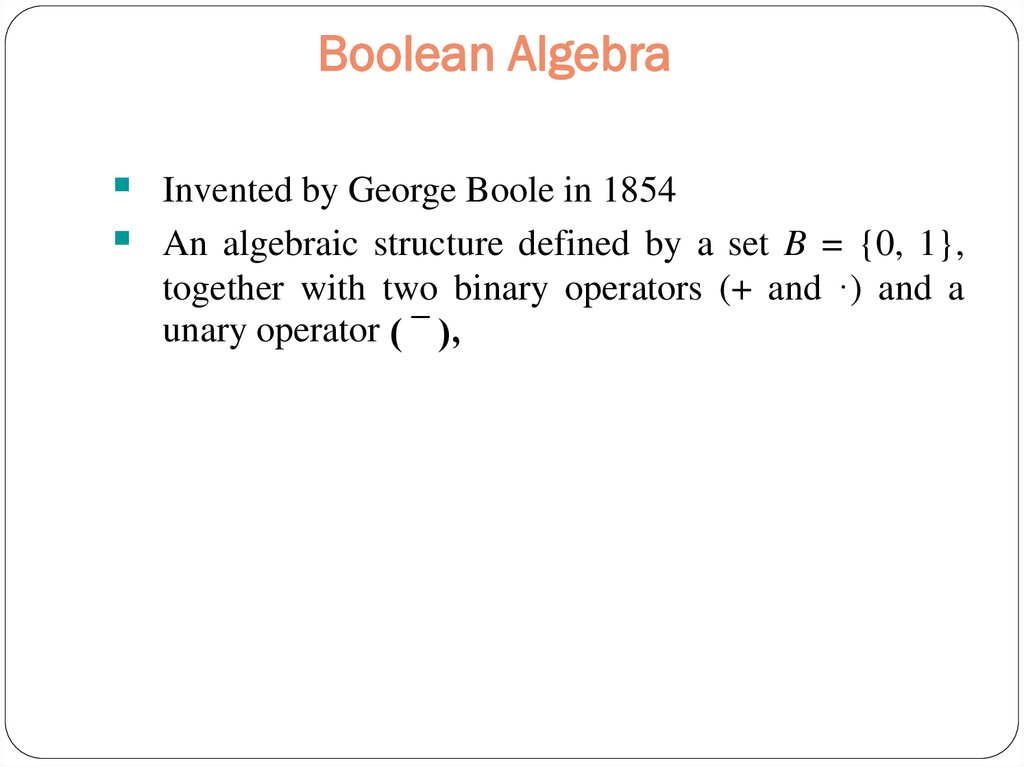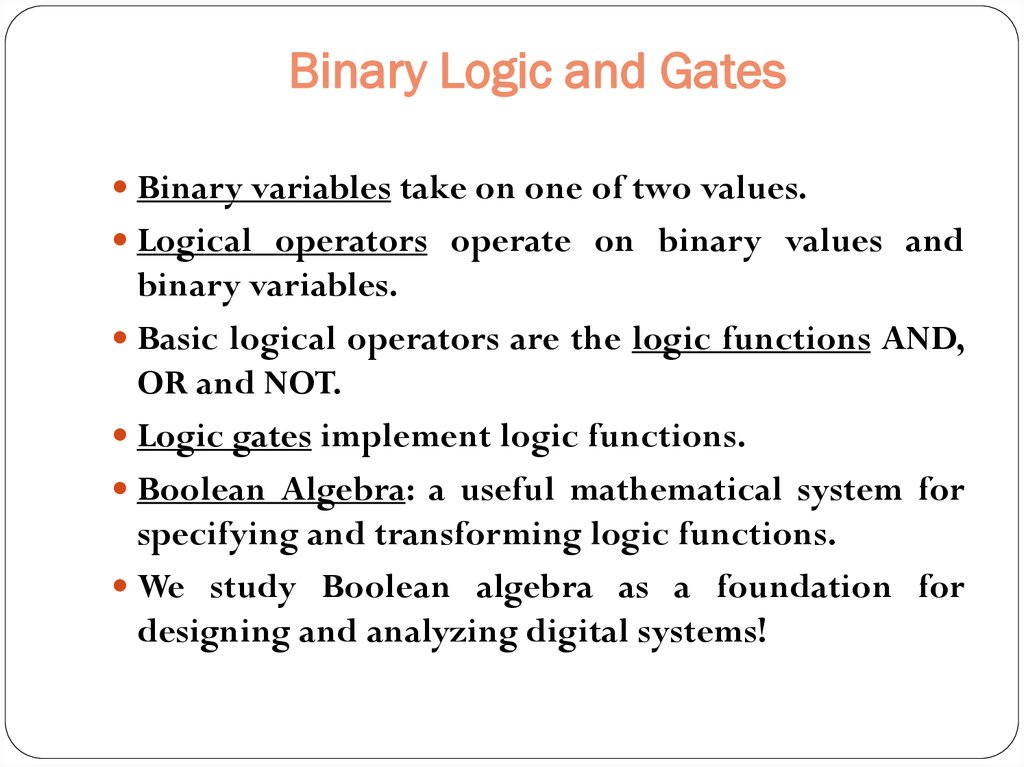Binary Variables

1. Binary Variables

Recall that the two binary values have different
names:
True/False
On/Off
Yes/No
1/0
We use 1 and 0 to denote the two values.

2. Boolean Algebra

Invented by George Boole in 1854
An algebraic structure defined by a set B = {0, 1},
together with two binary operators (+ and ·) and a
unary operator ( ¯ ),

3. Binary Logic and Gates

Binary variables take on one of two values.
Logical operators operate on binary values and
binary variables.
Basic logical operators are the logic functions AND,
OR and NOT.
Logic gates implement logic functions.
Boolean Algebra: a useful mathematical system for
specifying and transforming logic functions.
We study Boolean algebra as a foundation for
designing and analyzing digital systems!

4. Logic Gates

In the earliest computers, switches were opened
and closed by magnetic fields produced by
energizing coils in relays. The switches in turn
opened and closed the current paths.
Later, vacuum tubes that open and close current
paths electronically replaced relays.
Today, transistors are used as electronic switches that
open and close current paths.

5. Logical Operations

The three basic logical operations are:
AND
OR
NOT
AND is denoted by a dot (·).
OR is denoted by a plus (+).
NOT is denoted by an overbar ( ¯ ), a single quote
mark (') after, or (~) before the variable.

8. Produce a truth table l

In the BooleanAlgebra, verify using truth table that (X + Y)’ = X’Y’
In the Boolean Algebra, verify using truth table that X + XY = X

11. Problem 1

A system used 3 switches A,B and C; a combination of
switches determines whether an alarm, X, sounds:
If switch A or Switch B are in the ON position and if switch
C is in the OFF position then a signal to sound an alarm, X is
produced.
Convert this problem into a logic statement.

12. Problem 2

A nuclear power station has a safety system based on three inputs to a
logic circuit(network). A warning signal ( S = 1) is produced when
certain conditions in the nuclear power station occur based on these
three inputs
Input
T
P
W
Binary Values
1
0
1
0
1
0
Description of plant status
Temperature > 115 C
Temperature <= 115 C
Reactor pressure > 15 bar
Reactor pressure <= 15 bar
Cooling water > 120 litres / hour
Cooling water<=120 liters/hour
A warning signal (S=1) will be produced when any of the following
occurs.
Either (a) Temperature > 115 C and Cooling water <=120 litres/hour
or
(b) Temperature <=115 C and when Reactor pressure > 15 bar
or cooling water <= 120 litres/hour
Draw a logic circuit and truth table to show all the possible situations
when the warning signal (S) could be received.

15. Some Properties of Boolean Algebra

Boolean Algebra is defined in general by a set B that can have more
than two values
A two-valued Boolean algebra is also know as Switching Algebra. The
Boolean set B is restricted to 0 and 1. Switching circuits can be
represented by this algebra.
The dual of an algebraic expression is obtained by interchanging +
and · and interchanging 0’s and 1’s.
The identities appear in dual pairs. When there is only one identity
on a line the identity is self-dual, i. e., the dual expression = the
original expression.
Sometimes, the dot symbol ‘ ’ (AND operator) is not written when
the meaning is clear.

16. Dual of a Boolean Expression

Example: F = (A + C) · B + 0
dual F = (A · C + B) · 1 = A · C + B
Example: G = X · Y + (W + Z)
dual G = (X+Y) · (W · Z) = (X+Y) · (W+Z)
Example: H = A · B + A · C + B · C
dual H = (A+B) · (A+C) · (B+C)

17. Boolean Algebraic Proof – Example 1

A+A·B=A
Proof Steps
A+A·B
=A·1+A·B
= A · ( 1 + B)
=A·1
=A
(Absorption Theorem)
Justification
Identity element: A · 1 = A
Distributive
1+B=1
Identity element
Our primary reason for doing proofs is to learn:
Careful and efficient use of the identities and theorems of Boolean
algebra, and
How to choose the appropriate identity or theorem to apply to make
forward progress, irrespective of the application.

21. Simplification of Boolean Algebra

(A + B)(A + C) = A + BC
This rule can be proved as follows:
(A + B)(A + C) = AA + AC + AB + BC( Distributive law)
= A + AC + AB + BC ( AA = A)
= A( 1 + C) + AB + BC
(1 + C = 1)
= A. 1 + AB + BC
= A(1 + B) + BC
(1 + B = 1)
= A. 1 + BC
( A . 1 = A)
= A + BC

27. Simplification-Example

Using Boolean algebra techniques, simplify this expression:
AB + A(B + C) + B(B + C)
Step 1: Apply the distributive law to the second and third terms in
the expression, as follows:
AB + AB + AC + BB + BC
Step 2: Apply (BB = B) to the fourth term.
AB + AB + AC + B + BC
Step 3: Apply (AB + AB = AB) to the first two terms.
AB + AC + B + BC
Step 4: Apply (B + BC = B) to the last two terms.
AB + AC + B
Step 5: Apply (AB + B = B) to the first and third terms.
B+AC

0
1
0

1
1
0

35. Canonical Forms…..

Minterms and Maxterms
Sum-of-products (SOP) Canonical Form
Product-of-sum (POS) Canonical Form
Representation of Complements of Functions
Conversions between Representations

38. Minterms & Maxterms for 2 variables

Minterms & Maxterms for 2 variables

39. Minterms & Maxterms for 3 variables

Minterms & Maxterms for 3 variables

40. The Standard SOP Form

A standard SOP expression is one in which all the variables
in the domain appear in each product term in the
expression.
Example:
AB CD A B CD ABC D
Standard SOP expressions are important in:
Constructing truth tables
The Karnaugh map simplification method

41. Converting Product Terms to Standard SOP (example)

Convert the following Boolean expression into standard
SOP form:
AB C A B ABC D
AB C AB C ( D D ) AB CD AB CD
A B A B (C C ) A B C A B C
A B C ( D D ) A B C ( D D ) A B CD A B CD A B C D A B C D
AB C A B ABC D AB CD AB CD A B CD A B CD A B C D A B C D ABC D
8

44. Implementation of an SOP

X=AB+BCD+AC
AND/OR implementation
NAND/NAND implementation
A
B
A
B
B
C
D
B
C
D
A
C
X
A
C
X

45. The Standard POS Form

A standard POS expression is one in which all the
variables in the domain appear in each sum term in the
expression.
Example:
( A B C D )( A B C D)( A B C D)
Standard POS expressions are important in:
Constructing truth tables
The Karnaugh map simplification method

46. Converting a Sum Term to Standard POS (example)

Convert the following Boolean expression into standard
POS form:
( A B C )( B C D )( A B C D)
A B C A B C DD ( A B C D)( A B C D )
B C D B C D AA ( A B C D )( A B C D )
( A B C )( B C D )( A B C D)
( A B C D)( A B C D )( A B C D )( A B C D )( A B C D)